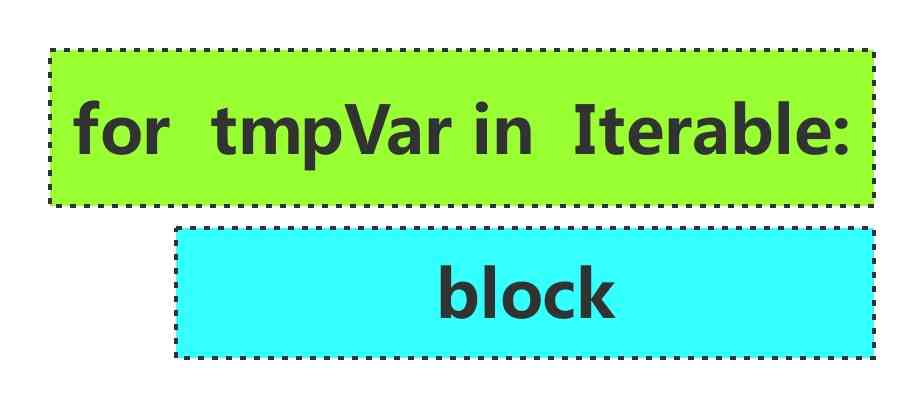# 嵌套循环## 1. 两层嵌套for循环

``````for i in range(3):
for j in range(3):
print(i, j)
``````

``````0 0
0 1
0 2
1 0
1 1
1 2
2 0
2 1
2 2
``````

``````for j in range(3):
print(i, j)
``````

``````j = 0, 进入循环体执行print(i, j)，此时i=0,j=0,输出结果是0 0
``````

``````j = 1, 进入循环体执行print(i, j)，此时i=0,j=1,输出结果是0 1
``````

``````j = 2, 进入循环体执行print(i, j)，此时i=0,j=2,输出结果是0 2
``````

3轮之后，内层循环结束，此时开始外层循环的第2轮，i=1，老规矩，进入循环体，而循环体里的代码还是

``````for j in range(3):
print(i, j)
``````

``````1 0
1 1
1 2
``````

``````2 0
2 1
2 2
``````

## 2. 3层嵌套循环

``````lst1 = [2, 1, 4, 6, 5]
lst2 = [3, 2, 7, 4]
lst3 = [4, 5, 2, 3]
``````

``````lst1 = [2, 1, 4, 6, 5]
lst2 = [3, 2, 7, 4]
lst3 = [4, 5, 2, 3]

for i in lst1:
for j in lst2:
for k in lst3:
if i + j + k == 10:
print((i, j, k))
``````

``````(2, 3, 5)
(2, 4, 4)
(1, 7, 2)
(1, 4, 5)
(4, 3, 3)
(4, 2, 4)
(4, 4, 2)
(6, 2, 2)
(5, 3, 2)
(5, 2, 3)
``````

## 3. 嵌套循环的终止

``````lst1 = [2, 7, 4, 6, 5]
lst2 = [3, 2, 7, 4]
``````

``````lst1 = [2, 7, 4, 6, 5]
lst2 = [3, 2, 7, 4]

for i in lst1:
for j in lst2:
if i + j == 10:
print((i, j))
break
``````

``````(7, 3)
(6, 4)
``````

``````lst1 = [2, 7, 4, 6, 5]
lst2 = [3, 2, 7, 4]

stop = False
for i in lst1:
for j in lst2:
if i + j == 10:
print((i, j))
stop = True
break

if stop:
break
``````QQ交流群: 211426309